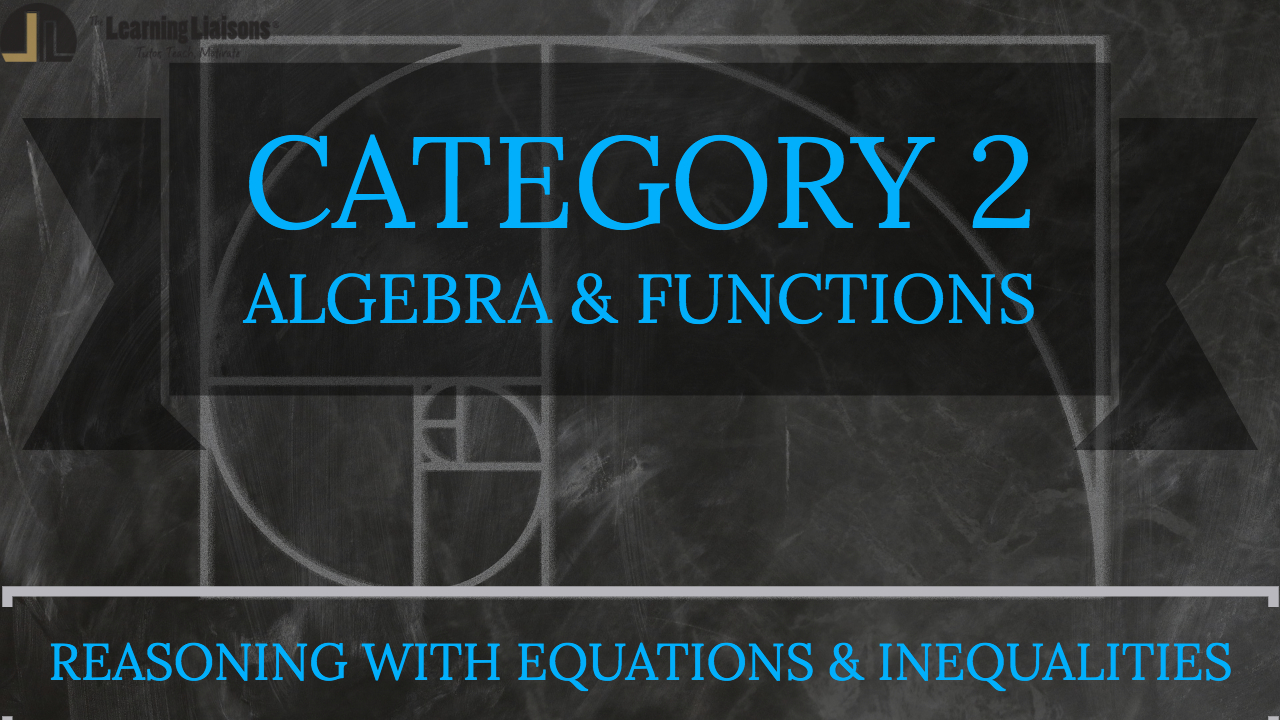# B. Reasoning with Equations and Inequalities

As found on the official ETS website, this Category details the following skills:

1. Understand the connections between proportional relationships, lines, and linear equations
2. Understand solving equations as a process of reasoning and explain the reasoning
3. Reason about and solve one-variable equations and inequalities
4. Solve equations and inequalities in one variable
5. Analyze and solve linear equations and pairs of simultaneous linear equations
6. Represent and solve equations and inequalities graphically

To better understand the fundamentals of these skills, try using the pedagogically sound Praxis Core Math Boot Camp by The Learning Liaisons. With hours of teaching content, practice sets for each of the 15 skills, and video solutions for each problem, you'll be in the best position to pass your Praxis Core Math test!